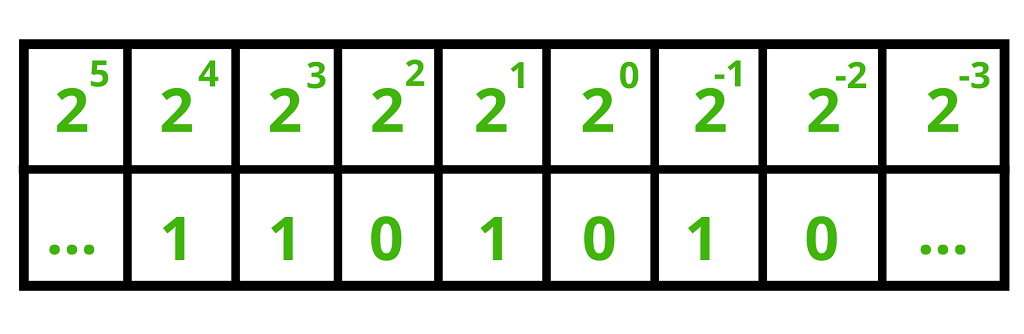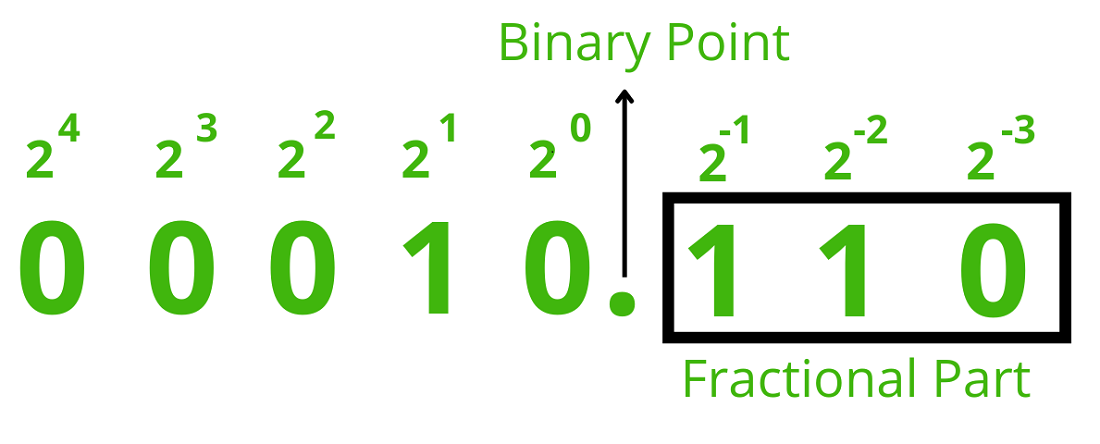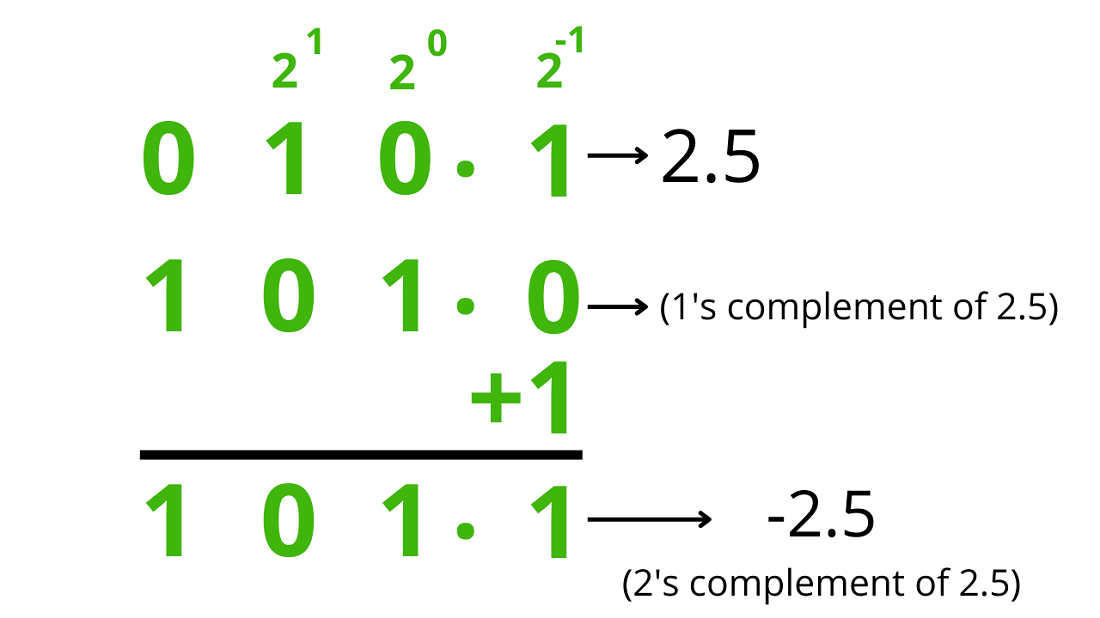GFG App
Open AppBrowser
Continue

Real numbers have a fractional component. This article explains the real number representation method using fixed points. In digital signal processing (DSP) and gaming applications, where performance is usually more important than precision, fixed point data encoding is extensively used.

The Binary Point: Fractional values such as 26.5 are represented using the binary point concept. The decimal point in a decimal numeral system and a binary point are comparable. It serves as a divider between a number’s integer and fractional parts.

For instance, the weight of the coefficient 6 in the number 26.5 is 100, or 1. The weight of the coefficient 5 is 10-1 or (5/10 = 1/2 = 0.5).

2 * 101 + 6 * 100 + 5 * 10-1 = 26.5

2 * 10 + 6 * 1 + 0.5 = 26.5

A “binary point” can be created using our binary representation and the same decimal point concept. A binary point, like in the decimal system, represents the coefficient of the expression 20 = 1. The weight of each digit (or bit) to the left of the binary point is 20, 21, 22, and so forth. The binary point’s rightmost digits (or bits) have weights of 2-1, 2-2, 2-3, and so on.

For illustration, the number 11010.12 represents the value:

11010.12

= 1 * 24 + 1 * 23 + 0 * 22 + 1 * 21 + 0* 20 + 1 * 2-1

= 16 + 8 + 2 + 0.5

= 26.5### Shifting Pattern:

When an integer is shifted right by one bit in a binary system, it is comparable to being divided by two. Since we cannot represent a digit to the right of a binary point in the case of integers since there is no fractional portion, this shifting operation is an integer division.

• A number is always divided by two when the bit pattern of the number is shifted to the right by one bit.
• A number is multiplied by two when it is moved left one bit.

### How to write Fixed Point Number?

Understanding fixed point number representation requires knowledge of the shifting process described above. Simply by implicitly establishing the binary point to be at a specific place of a numeral, we can define a fixed point number type to represent a real number in computers (or any hardware, in general). Then we will just use this implicit standard to express numbers.

Two arguments are all that are required to theoretically create a fixed point type:

1. Width of the number representation.
2. Binary point position within the number.

the notation fixed<w, b>, where “w” stands for the overall amount of bits used (the width of a number) and “b” stands for the location of the binary point counting from the least significant bit (counting from 0).

### Unsigned representation:

For example, fixed<8,3> signifies an 8-bit fixed-point number, the rightmost 3 bits of which are fractional.

Representation of a real number:

00010.1102
= 1 * 21 + 1 * 2-1 + 1 * 2-2

= 2 + 0.5 + 0.25

= 2.75### Signed representation:

Negative integers in binary number systems must be encoded using signed number representations. In mathematics, negative numbers are denoted by a minus sign (“-“) before them. In contrast, numbers are exclusively represented as bit sequences in computer hardware, with no additional symbols.

Signed binary numbers (+ve or -ve) can be represented in one of three ways:

1. Sign-Magnitude form
2. 1’s complement form
3. 2’s complement form

Sign-Magnitude form: In sign-magnitude form, the number’s sign is represented by the MSB (Most Significant Bit also called as Leftmost Bit), while its magnitude is shown by the remaining bits (In the case of 8-bit representation Leftmost bit is the sign bit and remaining bits are magnitude bit).

55 10 = 001101112

−55 10 = 101101112

1’s complement form: By complementing each bit in a signed binary integer, the 1’s complement of a number can be derived. A result is a negative number when a positive number is complemented by 1. Similar to this, complementing a negative number by 1 results in a positive number.

55 10 = 001101112

−55 10 = 110010002

2’s complement form: By adding one to the signed binary number’s 1’s complement, a binary number can be converted to its 2’s complement. Therefore, a positive number’s 2’s complement results in a negative number. The complement of a negative number by two yields a positive number.

55 10 = 11001000 + 1 (1’s complement + 1 = 2’s complement)

-55 10 = 11001001 2

### Fixed Point representation of negative number:

Consider the number -2.5, fixed<w,b> width = 4 bit, binary point = 1 bit (assume the binary point is at position 1). First, represent 2.5 in binary, then find its 2’s complement and you will get the binary fixed-point representation of -2.5.

2.5 10 = 0101 2

-2.5 10 = 1010 2 + 1 (1’s complement + 1 = 2’s complement)

-2.5 10 = 1011 2### 1’s complement representation range:

One bit is essentially used as a sign bit for 1’s complement numbers, leaving you with only 7 bits to store the actual number in an 8-bit number.

Therefore, the biggest number is just 127 (anything greater would require 8 bits, making it appear to be a negative number).

The least figure is likely to be -127 or -128 as well.

1’s complement:

127 = 01111111 : 1s complement is 10000000

128 = 10000000 : 1s complement is 01111111

We can see that storing -128 in 1’s complement is impossible (since the top bit is unset and it looks like a positive number)

The 1’s complement range is -127 to 127.

### 2’s complement representation range:

Additionally, one bit in 2’s complement numbers is effectively used as a sign bit, leaving you with only 7 bits to store the actual number in an 8-bit integer.

2’s complement:

127 = 01111111 : 2s complement is 10000001

128 = 10000000 : 2s complement is 10000000

we can see that we can store -128 in 2s complement.

The 2s complement range is -128 to 127.

### Advantages of Fixed Point Representation:

• Integer representation and fixed point numbers are indeed close relatives.
• Because of this, fixed point numbers can also be calculated using all the arithmetic operations a computer can perform on integers.
• They are just as simple and effective as computer integer arithmetic.
• To conduct real number arithmetic using fixed point format, we can reuse all the hardware designed for integer arithmetic.

### Disadvantages of Fixed Point Representation:

• Loss in range and precision when compared to representations of floating point numbers.

My Personal Notes arrow_drop_up